## Carry Counts

Hello all!

It’s been a while since I’ve been able to post, but I wanted to cover another Bloomsburg problem from 2018 for my programming club.  The problem description is this:

When calculating the sum of two numbers by hand, we first add the digits in the 1’s position, and if the result overflows (i.e., is greater than 9) then we carry the leftmost 1 in the result to the 10’s position. This process is repeated at the 10’s position, then at the 100’s position, and so on. For example, if we are calculating 1523 + 817 by hand, we write: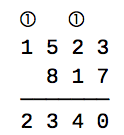In this case, there are two carries (shown in circles).

Write a program that prompts the user for two positive integers and outputs the number of carries that would occur when performing addition by hand.

The following test cases illustrate the required I/O format.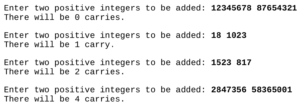We’ll begin this problem by defining our variables and taking in some input.  Our input will be the two integers we will be adding, and then we will be putting them into a list.

carries = 0
int1 = int(raw_input("Enter two positive integers to be added: "))
int2 = int(raw_input())
ints = [int1, int2]

Next up we need to define our algorithm we will be using.   For this problem we will be using a new function called zfill(). The zfill() function fills a string up with zeroes on the left side until a specific length is reached.  We’ll also be using the .max() method to find the maximum value of the list, and then run the len() method on it to get the max length.  Doing this we can fill up both integers until they are the same length, allowing me to then work backwords through them, adding each value from right to left and counting the carries for each value.

length = len(max(ints))
add2 = int2.zfill(length)

Then we’ll use a for loop to loop through the length of the integers, moving from right to left and adding each value.  If the summed value is greater than 9, then the value will have a carry.  We also need to create a variable called extra, which I will use as the actual carry, because in a case like 89+11 the carry from the ones value causes the tens value to also increase by one, so we must account for it.  Otherwise if the summed value is less than or equal to nine, we do nothing and set extra equal to zero.

for i in range(length):
extra = 1
carries += 1
extra = 0

After we’ve iterated through the integers, we just need to check how many carries there were and print our statements accordingly for grammatical correctness.

if carries == 1:
print("There will be 1 carry.")
elif carries <= 0:
print("There will be no carries.")
else:
print("There will be ", carries, " carries")

And now we have a working solution to Bloomsburg 2018 Problem 3, Carry Counts.  I hope this helps. 🙂

Thanks for reading and have a wonderful day!
~ Corbin

## PJAS and Some Catching Up

Hello world!

So if you’re just here for the technobabble about my PJAS project, skip down a little bit, otherwise I feel it necessary to explain where the heck I’ve been.   I managed to get myself stuck in a rut a few months ago, where I ended up staring at all my unfinished drafts and wondering why I even bother when I can’t finish a post (very bad thinking).  Pulling myself out of this rut was somewhat a revolutionary turn in my evolution towards growth mindset thinking.  To sum up the several sleepless nights, I essentially decided “improvement over time with crappy posts is better than having no consistency at all.”  So expect some weird consistency for a while. This also means I’ll have a few posts pop up on some old projects, but I feel better personally if I complete those posts rather than discard them.

Without further ado, onto the sciencey-things!  So back in February I competed in the regional competition for the Pennsylvania Junior Academy of Science, and won first place.  So then I competed again in May at the states competition, and won first place again!  (Woot woot!) … but what was the project you ask? Fantastic railroading question! I simulated and analyzed the spread of diseases using a set of differential equations.

To start I need to cover some basics of differential equations.  I have not taken a calculus course yet, so take what I say from here with skepticism, but from what I understand the differential equations I use give us the instantaneous slope for a given time on a graph, and this is essentially all you need to know to implement them into Python.  The equations we’ll be using are known as the SIR Model, it’s a model used for modeling disease spread and was proposed by Kermack and McKendrick in 1927.  The SIR Model:

(1)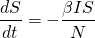(2)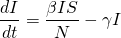(3)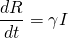The equation has a few key variables, N is the total population, S is susceptible stock, I is infected stock, and R is recovered stock.  Alongside these, we also have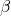and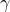is the transmission rate for the disease andis the recovery rate (usually 1 over the general recovery period).  In order to find the transmission rate for the disease, we need to know the recovery rate and the basic reproductive number (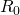).  Now in my research for this project, I couldn’t find much on how they calculate this number, but it seems to be generally available for most diseases online.  From the basic reproduction rate we can calculate our transmission rate using the following formula:

(4)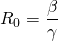(5)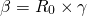That covers pretty much everything we need to know about the SIR Model, so onto the programming! For this project we’re gonna have to use SciPy, NumPy, and MatPlotLib.  We’ll start off by defining the many variables we’re gonna be using here.

# Total Population
N = 7406000
# Initial infected and recovered stock
I0, R0 = 1, 0
# (R_0)
R = 15
gamma = 1/21
beta = (gamma*R)
# Total susceptible stock
S0 = N - I0 - R0

The example I’m using here is a measles outbreak in Washington state, as it’s the same example I used when I competed at the state competition.  Next we create a vector of the initial conditions, we need to do this to pass it by SciPy’s Odeint function later in order to solve the differential equations.  We also create a time grid using numpy, this is to represent the total time simulated and be used in our final graphing task.

t = np.linspace(0, 160)
y0 = S0, I0, R0

Now we just need one final piece, the actual equations.  In order to pass them through SciPy it’s easiest if we have them inside their own function.  We just implement them as normal equations using the standard operators.

def SIR(y, t, N, beta, gamma):
S, I, R = y
dSdt = -beta * S * I / N
dIdt = beta * S * I / N - gamma * I
dRdt = gamma * I
return dSdt, dIdt, dRdt

After this we can integrate our model over our previously created time grid.  We do this using Odeint, which is one of SciPy’s integrate functions.  I’m not quite sure of the specifics of how this works because I haven’t taken calculus, but essentially it solves our equations for what we need.  First we initialize ret as an object of Odeint, then we run method T on it which solves it for what we need for our S, I, and R variables.

ret = odeint(SIR, y0, t, args=(N, beta, gamma))
S, I, R = ret.T

Now that we’ve solved our equation we just need to graph out our data using MatPlotLib.

fig = plt.figure()
ax.plot(t, S, 'r', label='Susceptible')
ax.plot(t, I, 'g', label='Infected')
ax.plot(t, R, 'b', label='Recovered')
ax.set_xlabel('Time (Days)')
ax.set_ylabel('Number of People (1,000,000s)')
ax.set_ylim(0,10000000)
legend = ax.legend()
legend.get_frame()
plt.show()

And finally, when we run our code we get nice graphs such as this:

It works! But as with all things, there are some problems.  The first is that the SIR Model I chose to use lacks something called vital dynamics.  Put simply vital dynamics are natural birth and death rates.  The second is that it doesn’t take into account many factors such as autoimmune diseases, dietary differences, and so many other things that can vary the data.  And third, any value we use for our recovery period or transmission rate will always be an average or a general number because diseases and humans are all different.

Overall this was one heck of a project, it was great fun to research into, and winning first place was a great bonus.  My slides from the competition are attached on the new “Slides” page on the site.  I’m working on many more projects this summer including some research with a college professor, so come back for that!

Thanks for reading and have a wonderful day!
~ Corbin

## Vowel Shifter

Hello all!

This week we have another practice problem for the Bloomsburg Competition coming up.  This problem is a vowel shifter and the description goes as follows:

Write a program that prompts the user for a sentence and modifies it by shifting each vowel like this:
• a→ e
• e→ i
• i→ o
• o→ u
• u→ a
In other words, each “a” in the original sentence becomes an “e”, each “e” in the original sentence becomes an “i”, and so on, and similarly for capital letters.

We’ll start this program off by creating two lists for each of our vowel sets. These will be called vowelsupper and vowelslower.

vowels = ["a", "e", "i", "o", "u", "a"]
vowelsupper = ["A", "E", "I", "O", "U", "A"]

Next we need to grab our input from the user using phrase = str(raw_input("Enter a sentence.\n")) (Sidenote: The \n at the end of the sentence is an escape operator that just starts a new line.).  Next we need to create a way to iterate through our users input to find and replace vowels with our new shifted vowels.  We do this using a for loop.  A for loop is just a loop that repeats a set number of times and often is used to create a changing variable for the program. Inside this loop we want to use a conditional statement to check if each letter in the phrase is a vowel, and if it is a vowel we want to check if it is upper or lower case. After doing this we will shift the vowel and add the new vowel to our shifted phrase. Then we just repeat this process until we have iterated through the entire original string.

for i in range(len(phrase)):
if phrase[i] in vowelslower or phrase[i] in vowelsupper:
if phrase[i].islower():
shift += vowelslower[vowelslower.index(phrase[i])+1]
else:
shift += vowelsupper[vowelsupper.index(phrase[i])+1]
else:
shift += phrase[i]

Now we have all the main components needed to create our program.  After combining them all together our final code will look like this:

vowelslower = ["a", "e", "i", "o", "u", "a"]
vowelsupper = ["A", "E", "I", "O", "U", "A"]
shift = ""
phrase = str(raw_input("Enter a sentence.\n"))
for i in range(len(phrase)):
if phrase[i] in vowelslower or phrase[i] in vowelsupper:
if phrase[i].islower():
shift += vowelslower[vowelslower.index(phrase[i])+1]
else:
shift += vowelsupper[vowelsupper.index(phrase[i])+1]
else:
shift += phrase[i]
print(shift)

And now we have a working solution for Problem #2!  This solution is posted on my GitHub as well.

Thanks for reading and have a wonderful day!
~ Corbin

## Okapi and Preparing for the Bloomsburg Competition

Hello all!

I’ve been on a bit of a ‘hiatus’ lately, I’ve been busy with life things and haven’t had a chance to work on any posts here.  But a quick update, I won first place at regionals for the Pennsylvania Junior Academy of Science so I’m going to states in May and I’ll be making a post on that project soon.  I’ve also been preparing the programming club at my school for an upcoming competition at Bloomsburg University where we will be competing.  Because of this we have been doing practice problems and so I will be posting and explaining my solutions to them here.

Our first practice problem is called Okapi.  The problem description goes as follows:

The game of Okapi is played by rolling three dice. A payout in dollars is determined by the rolled numbers according to the following rule:

• If the three numbers are the same, the player wins the sum of those three numbers.
• If only two of the numbers are the same, the player wins the sum of the two equal numbers.
• For three different numbers, the player wins nothing.

Write a program that prompts the user for three dice rolls and outputs the payout.

We need to begin this problem by taking user input using rolls = input("Enter dice rolls: ") which prompts the user for input and sets rolls equal to their input. Next we need to parse out their answer into three separate rolls, this is rather easy and just a matter of indexing the user input. In order to do this we just need to create variables for each roll and then set them to the correct index of rolls using the following code: roll_one, roll_two, roll_three = int(rolls), int(rolls), int(rolls). Now that we have our rolls assigned we just need to use a bunch of conditional statements to determine the output.  Our final code will look like this:

def okapi():
rolls = input("Enter dice rolls: ")
roll_one, roll_two, roll_three = int(rolls), int(rolls), int(rolls)
if roll_one == roll_two and roll_two == roll_three:
print("The payout is $", roll_one*3, ".") elif roll_one == roll_two: print("The payout is$", roll_one+roll_two, ".")
elif roll_two == roll_three:
print("The payout is $", roll_two+roll_three, ".") elif roll_one == roll_three: print("The payout is$", roll_one+roll_three, ".")
else:
print("The payout is \$0.")

And now we have a working solution to problem #1!  Another solution can be found on my GitHub, it’s the same premise but just less readable.  I’ll most likely be posting around weekly again soon.

Thanks for reading and have a wonderful day!
~ Corbin

## Diving into Machine Learning

Hello all!

So lately I’ve been messing with machine learning because I’ve always been interested in it and it’s just very cool and interesting to me.  I’d like to talk a bit about what I’ve been doing and struggling with and show some examples. I will be working with scikit learn for Python, and it comes with 3 datasets. Iris and Digits are for classification and Boston House Prices are for regression. Simply put classification is identifying something like a handwritten number as the correct number it is and regression is essentially finding a line of best fit for a dataset.  I still have a lot to learn about sklearn and machine learning in general, but I find it really interesting nonetheless and thought you guys would too.

So my code begins with the import of a bunch of libraries.  The only ones I use in my example here are sklearn and matplotlib, the others are simply either dependencies or libraries I plan to use in the future.

import sklearn
from sklearn import datasets
import numpy as np
import pandas as pd
import quandl
import matplotlib.pyplot as plt
from sklearn import svm

In this import, sklearn is the main library I’m using to fit my data and predict things, sklearn.datasets comes with the 3 base datasets Iris Digits and Boston Housing Prices.  I don’t know much about sklearn.svm, but I do know that it is the support vector machine which essentially separates our inputted data and runs our actual machine learning, so when we input testing data it can determine what number we have written. Numpy is a science / math library that adds support for larger multidimensional arrays and matrices. Pandas is a library for data analysis. Quandl is a financial library that lets me pull a lot of data that I can use for linear regression in the future. And matplotlib and it’s sub-library pyplot allow me to output the handwriting data.
So far my code for the recognition looks like this:

clf = svm.SVC(gamma=0.001, C=100)
clf.fit(digits.data[:-1], digits.target[:-1])
clf.predict(digits.data[-1:])
plt.figure(1, figsize=(3, 3))
plt.imshow(digits.images[-1], cmap=plt.cm.gray_r, interpolation='nearest')
plt.show()

Although my understanding is rudimentary, I can explain a little bit of what this does. Clf is our estimator which is the actual machine that is learning, and that is what we pass out training data through with clf.fit().  Clf.fit() lets us pass data into the svm that we made clf off of, and it trains our machine to know what the numbers should look like.  I am passing all digits except for the last one through this function, because we will be testing with the last one.  We then pass a digit through clf using clf.predict(),  which passes data for a know handwritten digit, 8, through clf.  Our object clf then outputs the text <code>array()</code> which means that it has predicted our inputted number as 8.  If we print out digits.target[-1:] we can see it and determine if it was correct. We do this using out 3 lines from matplotlib that create the figure, print it, and then show it. The figure we get is this:It’s a very low resolution, but it’s an 8! I think that this is brilliant, and I definitely need to learn more about what is happening here with my code. Machine learning is very cool and I definitely need to mess with it more and learn more.  So far I’m learning some of the basic elements like how to fit and predict things, how training and testing sets work, and a lot of the vocabulary that is used when talking about machine learning.  I can now actually talk about things like supervised and unsupervised learning, or classification and regression methods.  Along with this, I’m also learning more about other libraries like matplotlib, and how to write more pythonic (readable) code.  For anyone who wants to try this themselves, there’s a lot of really cool stuff online, but I’m using some of the resources from hangtwenty‘s GitHub repo dive-into-machine-learning.  It can be found here: https://github.com/hangtwenty/dive-into-machine-learning Hopefully by my next post I will have created a basic understanding of linear regression and I can create some cool examples using it, and in my next post I will attempt to give my explanation on how fitting, predicting, and training actually works.

Thanks for reading and have a wonderful day!
~ Corbin

## Mindsets and Prime Factorization

Hello all!

It’s been a while since I last posted, apologies. I’ll be doing another Project Euler problem today.

Problem 3:

The prime factors of 13195 are 5, 7, 13 and 29.

What is the largest prime factor of the number 600851475143 ?

I’d like to begin by saying that I spent way too long on this problem improving my code after I had already solved it. But this is a very good mindset to have when you are trying to become better at something. Due to the increased complexity of this problem, it is a good idea to write an algorithm for it, and I will likely do this for all future problems.

def largest_prime(n)
Iterate through each number up until the square root of n
If the iterable is prime and the modulo of the base and iterable is 0:
Append the iterable to some empty list
print the [-1] element of the list, and this is the largest prime

I decided on an algorithm where I will check every number’s divisibility and ‘prime-ness’ up until the square root of the original number. This works because you can have no prime factor larger than the square root of the number. Next, I add every valid number to some list, and then I go back and pick out the largest one from the list and this is the answer’s problem. Now I need to turn this into working code, and then from there, I can improve it.

def largest_prime(base):
primes = []
root = math.sqrt(base)
for i in range(int(root+1)):
if is_prime(i) == True and base%i == 0:
primes.append(i)
prime = primes[-1]
print(prime)
print(primes)

In theory, this code should work but now I need to make a function is_prime(n) that allows me to check if a number is prime for some boolean value. I’ll do this by checking every number up until the square root for divisibility, and if I find no divisibility other than 1 and the number itself then I will declare the number as prime.

def is_prime(n):
if n <= 1:
return False
for i in range(2, (int(math.sqrt(n))+1)):
if n%i == 0:
return False
return True

Although all of this code works, it is very inefficient. If I insert a timer using from time import * my code takes an average of roughly 7 seconds to run. I would like to cut this down to 1 second or less in any way I can. One way I can optimize the code is by removing my use of lists, but this only saves me ~1 second. This is where I’ll introduce recursion into my programming repertoire. Recursion is when you have a function that calls on itself repeatedly until the task is done. When the task is done the look breaks and returns your answer.

In order to do this problem using recursion, I have to redefine my algorithm. Instead of using another function is_prime(n) I’ll be taking each number, finding the lowest common divisor. I will then take this new number, (n/lcd), and perform the same method on it until I can no longer be divided and this final number is our largest prime factor.

def largest_prime(base):
start = clock()
for i in range(2, int(math.sqrt(base)+1)):
if base % i == 0:
return largest_prime(base/i)
print(base)
end = clock()
print(end-start)

This is my final piece of code and it takes ~0.0008 seconds to run. I am very happy with the results as the code is simple and condensed. I’d like to end this post by talking about mindsets and why they matter. When you’re trying to get better at something you should realize that your abilities are not as fixed as you think they are. I see this problem a lot in my high school, kids in my Pre-Calculus class often complain that they are bad at math but then when they like a topic we are learning in the class they do extremely well at it because they enjoy it and put effort into it. A good analogy of this is someone learning to play an instrument. When you’re practicing hitting higher notes on a trumpet you don’t squeak a note and say “I’m bad at this and I should just give up trumpet” you instead say “Okay, I need to put faster air through the trumpet” and you try again, so why not do this with other things you are trying to get good at? If you would like to know some more about mindsets I highly recommend Mindset: The New Psychology of Success by Carol Dweck. It details lots of information and research about growth mindsets and how they can improve your life.

Thanks for reading and have a wonderful day!
~ Corbin

## Functions in Programming, List Comprehension, and Even Fibonacci Numbers

Hello all!

Today I’ll be doing another Project Euler problem.

Problem 2:

Each new term in the Fibonacci sequence is generated by adding the previous two terms. By starting with 1 and 2, the first 10 terms will be:

1, 2, 3, 5, 8, 13, 21, 34, 55, 89, …

By considering the terms in the Fibonacci sequence whose values do not exceed four million, find the sum of the even-valued terms.

There are many ways to do this problem, but I’ve chosen to create a series of functions that I can run to get Fibonacci numbers until some upper bound, and then the sum of even numbers in a list. I find that abstracting calculations like these into functions is a very easy way to make programs feel and look more organized as well as increasing reusability and convenience. A good example would be that I may need a Fibonacci sequence in a future problem, and using a function like this allows me to just paste this code into a future program and I can recall on it using fib(max) when needed. I’m going to begin by creating a function to find the Fibonacci numbers up to some upper bound. I’ll create the function fib(max) where max is the upper bound of the number we’ll be getting from the Fibonacci sequence. The best way I can come up with to create a Fibonacci sequence is to create a list and use fib[-1] and fib[-2] to grab the latest two numbers in the sequence. Next, I’ll add in a while loop that iterates through the Fibonacci sequence and places the numbers into the list fib[ ], to create the next Fibonacci number. This loop then breaks when we hit the upper bound we placed earlier. After this the function will return the newly filled list fib[ ].

def fib(max):
fib = [1, 2]
while(int(fib[-1] + fib[-2]) <= max):
fib.append(int(fib[-1]+fib[-2]))
return fib

Next, I need to create a function that will add the even numbers of a list together. I’ll call this function even_sum(list) and to create it I’m going to use something new I learned called List Comprehension. You can learn more about List Comprehension here but I’ll provide a quick overview. List Comprehension allows a smaller and more efficient way to create a list by placing a for loop inside of square brackets. We can also place operators such as if statements inside the brackets. Using this new tool my next function will look like this:

def even_sum(list):
return sum([i for i in list if i%2==0])

Now that we have both of our functions we can just combine the two and run them through each other like so:

even_sum(fib(4000000))

This now returns us with the sum of all even Fibonacci numbers up until 4 million!

Thanks for reading and have a wonderful day!
~ Corbin

I would like to give special thanks to Christian Ferko for teaching me about List Comprehension.

## Intro and Multiples of 3 and 5

Hello all!

I’ll insert a small introduction here. Welcome to my blog, my name is Corbin Frisvold and I’m a student interested in furthering my passions for Computer Science, Mathematics, and several other fields. Here my posts will primarily consist of my exploration into becoming a better programmer, but I will likely post other things around on the blog. Anyways, onto my first post!

Today I’m going to be doing a problem from Project Euler.
Problem 1:

If we list all the natural numbers below 10 that are multiples of 3 or 5, we get 3, 5, 6 and 9. The sum of these multiples is 23.

Find the sum of all the multiples of 3 or 5 below 1000.

I find it useful to just dive into the code for a small problem like this. We know we want a for loop iterating through 1000 and checking each number’s divisibility by 3 or 5. What I come out with is this:


sum = 0
for i in range(1000):
if i % 3 == 0:
sum += i
elif i % 5 == 0:
sum += i
print(sum)


What this code does is it creates a variable sum that will be used to store the sum of all our valid multiples. Then we use a for loop increment through all numbers between 1 and 1000. After running the program will print the output. Running this we get sum = 233168. And now we have solved Problem 1! My intention with post frequency is to post as much as I can, but I will attempt to keep a minimum frequency of 1 or 2 posts a week.

Thanks for reading and have a wonderful day!
~ Corbin